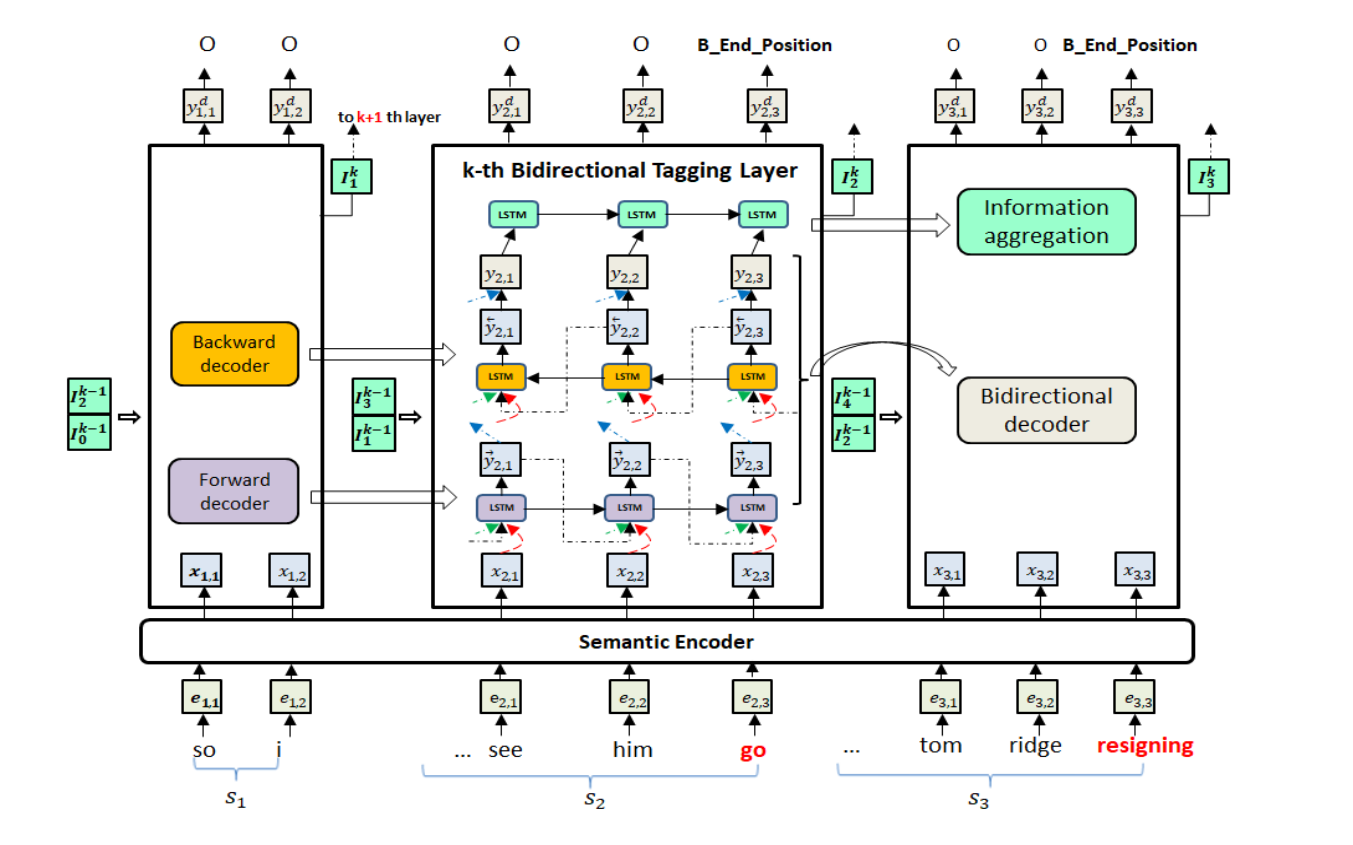# MLBiNet：跨句的语义信息提取

### MLBiNet: A Cross-Sentence Collective Event Detection Network

zjunlp/DocED: Source code for the ACL 2021 paper "MLBiNet: A Cross-Sentence Collective Event Detection Network ".

Seq2Seq 多层双向网络 捕捉多个不同事件。四个部分：语义编码器、双向解码器、信息聚合模块、堆叠式多元双向标记层

a) 独立的编码器模块在融合句子级和文档级语义信息方面具有灵活性;

b) RNN解码器模型的上下文向量和当前状态的学习捕捉：可以捕获序列事件标签相关性，将预测的标签向量作为预测 t 符号的输入

ED任务解码器的词汇表不是单词，而是事件类型的集合

#### 语义编码器

word embedding + NER type embedding -> e_t

LSTM + self-attn ->h_t^a

\mathbf x_t = [\mathbf h_t^a; \mathbf e_t]

#### 双向解码器

\begin{gathered}
\overrightarrow{\mathbf{s}}_{t}=f_{\mathrm{fw}}\left(\overrightarrow{\mathbf{y}}_{t-1}, \overrightarrow{\mathbf{s}}_{t-1}, \mathbf{x}_{t}\right) \\
\overrightarrow{\mathbf{y}}_{t}=\tilde{f}\left(W_{y} \overrightarrow{\mathbf{s}}_{t}+b_{y}\right)
\end{gathered}

\begin{gathered}
\overleftarrow{\mathrm{s}}_{t}=f_{\mathrm{bw}}\left(\overleftarrow{\mathbf{y}}_{t+1}, \overleftarrow{\mathrm{s}}_{t+1}, \mathrm{x}_{t}\right) \\
\overleftarrow{\mathbf{y}}_{t}=\tilde{f}\left(W_{y} \overleftarrow{\mathrm{s}}_{t}+b_{y}\right)
\end{gathered}

\tilde{\mathbf{I}}_{t}=\overrightarrow{\operatorname{LSTM}}\left(\tilde{\mathbf{I}}_{t-1}, \mathbf{y}_{t}\right)

#### 多层双向网络

\begin{gathered}
\overrightarrow{\mathbf{s}}_{t}=f_{\mathrm{fw}}\left(\overrightarrow{\mathbf{y}}_{t-1}^{k}, \overrightarrow{\mathbf{s}}_{t-1}, \mathbf{x}_{t}, \mathbf{I}_{i-1}^{k-1}, \mathbf{I}_{i+1}^{k-1}\right) \\
\overleftarrow{\mathbf{s}}_{t}=f_{\mathrm{bw}}\left(\overleftarrow{\mathbf{y}}_{t+1}, \overleftarrow{\mathbf{s}}_{t+1}, \mathbf{x}_{t}, \mathbf{I}_{i-1}^{k-1}, \mathbf{I}_{i+1}^{k-1}\right) \\
\overrightarrow{\mathbf{y}}_{t}^{k}=\tilde{f}\left(W_{y} \overrightarrow{\mathbf{s}}_{t}+b_{y}\right) \\
\overleftarrow{\mathbf{y}}_{t}^{k}=\tilde{f}\left(W_{y} \overleftarrow{\mathbf{s}}_{t}+b_{y}\right) \\
\mathbf{y}_{t}^{k}=\left[\overrightarrow{\mathbf{y}}_{t}^{k} ; \overleftarrow{\mathbf{y}}_{t}^{k}\right]
\end{gathered}0 评论